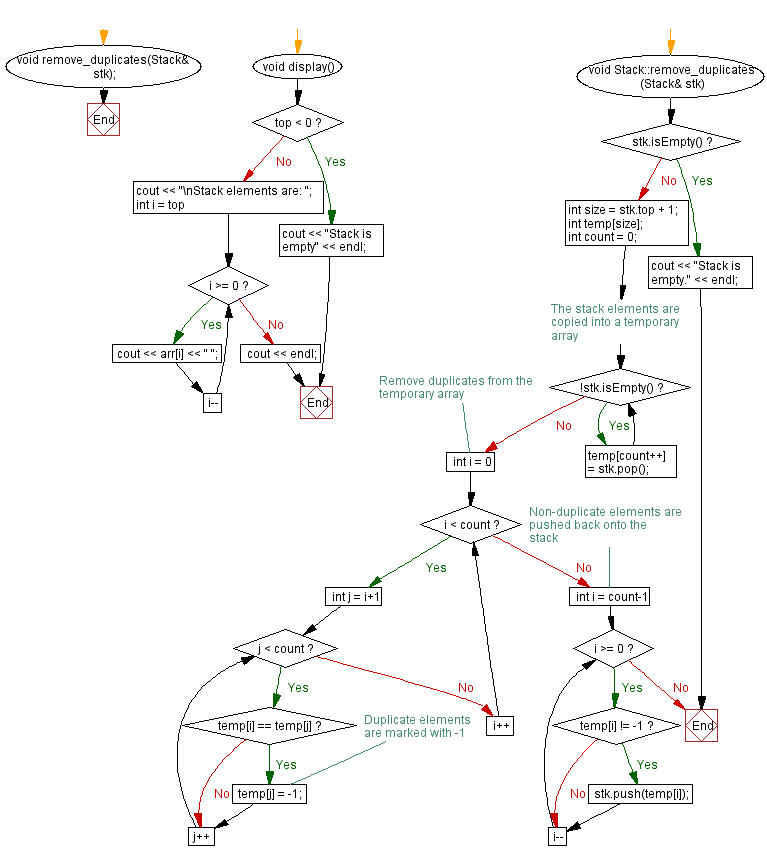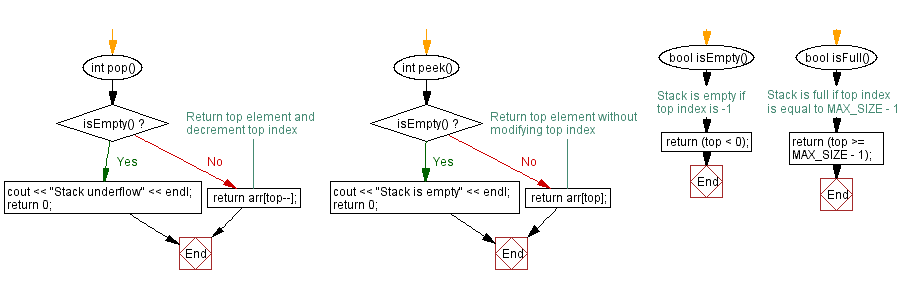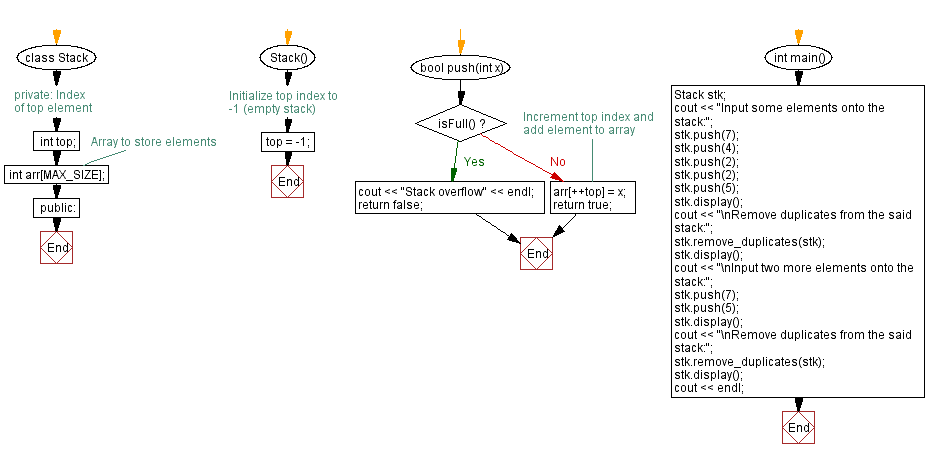﻿ C++ Remove duplicates from a stack using arrays

# C++ Stack Exercises: Remove duplicates from a stack using arrays

## C++ Stack: Exercise-12 with Solution

Write a C++ program to remove duplicates from a stack using arrays.

Test Data:
Input some elements onto the stack:
Stack elements are: 5 2 2 4 7
Remove duplicates from the said stack:
Stack elements are: 5 2 4 7

Sample Solution:

C++ Code:

``````#include <iostream>

using namespace std;
#define MAX_SIZE 15 // Maximum size of stack

class Stack {
private:
int top; // Index of top element
int arr[MAX_SIZE]; // Array to store elements
public:
Stack() {
top = -1; // Initialize top index to -1 (empty stack)
}

bool push(int x) {
if (isFull()) {
cout << "Stack overflow" << endl;
return false;
}
// Increment top index and add element to array
arr[++top] = x;
return true;
}

int pop() {
if (isEmpty()) {
cout << "Stack underflow" << endl;
return 0;
}
return arr[top--];
}

int peek() {
if (isEmpty()) {
cout << "Stack is empty" << endl;
return 0;
}
return arr[top];
}

bool isEmpty() {
// Stack is empty if top index is -1
return (top < 0);
}

bool isFull() {
// Stack is full if top index is equal to MAX_SIZE - 1
return (top >= MAX_SIZE - 1);
}

void display() {
if (top < 0) {
cout << "Stack is empty" << endl;
return;
}
cout << "\nStack elements are: ";
for (int i = top; i >= 0; i--)
cout << arr[i] << " ";
cout << endl;
}

void remove_duplicates(Stack& stk);
};

void Stack::remove_duplicates(Stack& stk) {
if (stk.isEmpty()) {
cout << "Stack is empty." << endl;
return;
}

int size = stk.top + 1;
int temp[size];
int count = 0;

//The stack elements are copied into a temporary array
while (!stk.isEmpty()) {
temp[count++] = stk.pop();
}

// Remove duplicates from the temporary array
for (int i = 0; i < count; i++) {
for (int j = i+1; j < count; j++) {
if (temp[i] == temp[j]) {
//Duplicate elements are marked with -1
temp[j] = -1;
}
}
}

//Non-duplicate elements are pushed back onto the stack
for (int i = count-1; i >= 0; i--) {
if (temp[i] != -1) {
stk.push(temp[i]);
}
}
}

int main() {
Stack stk;
cout << "Input some elements onto the stack:";
stk.push(7);
stk.push(4);
stk.push(2);
stk.push(2);
stk.push(5);
stk.display();
cout << "\nRemove duplicates from the said stack:";
stk.remove_duplicates(stk);
stk.display();
cout << "\nInput two more elements onto the stack:";
stk.push(7);
stk.push(5);
stk.display();
cout << "\nRemove duplicates from the said stack:";
stk.remove_duplicates(stk);
stk.display();
cout << endl;
}
``````

Sample Output:

```Input some elements onto the stack:
Stack elements are: 5 2 2 4 7

Remove duplicates from the said stack:
Stack elements are: 5 2 4 7

Input two more elements onto the stack:
Stack elements are: 5 7 5 2 4 7

Remove duplicates from the said stack:
Stack elements are: 5 7 2 4
```

Flowchart:CPP Code Editor: